# ISEE Lower Level Math : Geometry

## Example Questions

1 2 33 34 35 36 37 38 39 41 Next →

### Example Question #1 : How To Find Length Of A Line

What is the distance between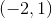and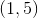?Explanation:

The distance formula is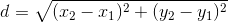.

Let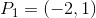and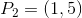.

Plug these points into the distance formula: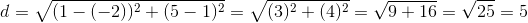### Example Question #2 : How To Find Length Of A Line

If a line that is 13 inches in length starts at the point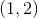, which of the following is NOT a possible end point?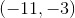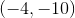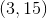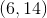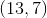Explanation:

The distance formula is given by.

Square both sides: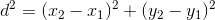Now, let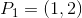, and let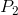be the end point we are looking for.

Pluginto the squared distance formula and solve for: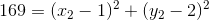Alternatively, you can plug in the answer choices and see which point makes the above equation untrue.  The pointmatches this description and is therefore the correct answer.

### Example Question #1332 : Isee Lower Level (Grades 5 6) Mathematics Achievement

How long is a line segment on the number line if it goes fromto?Explanation:

To find the length of a line on the number line when one point is negative and the other is positive, you must the the distances of both from zero.isspaces from zero where is the other point is just.  So the distance is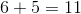.

### Example Question #3 : How To Find Length Of A Line

What is the distance between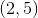and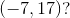Explanation:

The distance formula is.

Let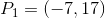and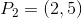: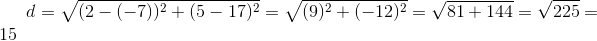1 2 33 34 35 36 37 38 39 41 Next →

### All ISEE Lower Level Math Resources